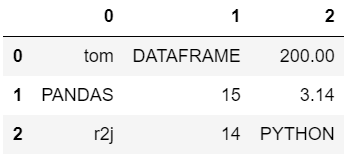# Extract all capital words from Dataframe in Pandas

In this article, we are to discuss various methods to extract capital words from a dataframe in the pandas module. Below is the dataframe which is going to be used to depict various approaches:

## Python3

 `# Import pandas library` `import` `pandas `   `# Create dataset` `data ``=` `[[``'tom'``, ``'DATAFRAME'``, ``'200.00'``],` `        ``[``'PANDAS'``, ``15``, ``3.14``],` `        ``[``'r2j'``, ``14``, ``'PYTHON'``]]`   `# Create the pandas DataFrame` `df ``=` `pandas.DataFrame(data)`   `# Display dataframe` `df`

Output:Method #1: Using ord() method in an explicit function

Create an explicit function to check if the string argument passed contains all capital characters or not. In the function check if each character is a capital letter or not by using their ASCII value. Now use that explicit function on each element of the dataframe to identify capital words and display them.

## Python3

 `# Import pandas library` `import` `pandas`     `# explicit function to check if string` `# contains only uppercase characters` `def` `findCap(s):` `    ``for` `ele ``in` `str``(s):` `        ``if` `ord``(ele) < ``65` `or` `ord``(ele) > ``90``:` `            ``return` `0` `    ``return` `1`     `# Create dataset` `data ``=` `[[``'tom'``, ``'DATAFRAME'``, ``'200.00'``],` `        ``[``'PANDAS'``, ``15``, ``3.14``],` `        ``[``'r2j'``, ``14``, ``'PYTHON'``]]`   `# Create the pandas DataFrame` `df ``=` `pandas.DataFrame(data)`     `# access each element in` `# the dataframe` `for` `i ``in` `range``(df.shape[``1``]):` `    ``for` `ele ``in` `df[i]:`   `        ``# call explicit function` `        ``if` `findCap(ele):` `            ``print``(ele)`

Output:

```PANDAS
DATAFRAME
PYTHON```

Method #2: Using str() and isupper() methods

Access each element of the dataframe and convert each element into a string using str(), after that apply isupper() method on each element. Extract the capital words from the dataframe and display them.

## Python3

 `# Import pandas library` `import` `pandas `     `# Create dataset` `data ``=` `[[``'tom'``, ``'DATAFRAME'``, ``'200.00'``],` `        ``[``'PANDAS'``, ``15``, ``3.14``],` `        ``[``'r2j'``, ``14``, ``'PYTHON'``]]`   `# Create the pandas DataFrame` `df ``=` `pandas.DataFrame(data)`     `# access each element in the dataframe` `for` `i ``in` `range``(df.shape[``1``]):` `   ``for` `ele ``in` `df[i]:` `       `  `       ``# use isupper() ` `       ``if` `str``(ele).isupper():` `            ``print``(ele)`

Output:

```PANDAS
DATAFRAME
PYTHON```

Method #3: Using str() method and regex module

Access each element of the dataframe and convert each element into a string using str(), after that apply the regex to extract the capital words from the dataframe and display them.

## Python3

 `# Import required modules` `import` `re` `import` `pandas `     `# Create dataset` `data ``=` `[[``'tom'``, ``'DATAFRAME'``, ``'200.00'``],` `        ``[``'PANDAS'``, ``15``, ``3.14``],` `        ``[``'r2j'``, ``14``, ``'PYTHON'``]]`   `# Create the pandas DataFrame` `df ``=` `pandas.DataFrame(data)`     `# access each element in the dataframe` `for` `i ``in` `range``(df.shape[``1``]):` `   ``for` `ele ``in` `df[i]:` `        ``if` `bool``(re.match(r``'\w*[A-Z]\w*'``, ``str``(ele))): ` `              ``print``(ele)`

Output:

```PANDAS
DATAFRAME
PYTHON```

Time Complexity: O(n*n)
Auxiliary Space: O(n), where n is length of list.

Whether you're preparing for your first job interview or aiming to upskill in this ever-evolving tech landscape, GeeksforGeeks Courses are your key to success. We provide top-quality content at affordable prices, all geared towards accelerating your growth in a time-bound manner. Join the millions we've already empowered, and we're here to do the same for you. Don't miss out - check it out now!

Previous
Next